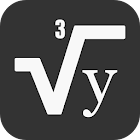MATHS FORMULA REFERENCE FREE

All Android applications categories

All Android games categories# MATHS FORMULA REFERENCE FREE

by: 290 8.4

8.4 Users
rating

## Screenshots

Description

A complete guide to maths formulas and concepts which is helpful in competitive like MBA , CAT , GMAT etc and curriculum exams .

The Complete Reference of Maths Formulas covering the following topics

* Trigonometry Formulas
Right Angled Definition
Pythagoras Theorem
Tangent Identitites
Even Odd Formulas
Multi angle formulas
Double Angle Formulas
Inverse Trigonometric Functions
Trigonometric Ratios

*Geometry Formulas
Basics and Definitions
Triangles
Areas and Circumference
Volumes and Surface Area

*Number System Formulas
Remainder
Unit Digit
Last 2 Digits

*Divisibility Rules
*Algebra Formulas
*Exponents Formulas
*Logarithms Formulas
*Progressions Formulas
*Squares , cubes and roots Formulas

This maths formulas collection is useful for MBA exams , competitive and even curriculum exam .

It lists out all the important maths formulas/topics in Algebra, Geometry, Trigonometry. Regular review of these maths formulas and concepts will definitely help improve your grades.

Write us at gamesnapps4u@gmail.com for suggestions and feedback for improvements.